Module Histo
opals::IHisto

# Aim of module

Derives histograms and descriptive statistics (min, max, mean, r.m.s, etc.) for ODM or grid/raster data sets and stores the results graphically (SVG) and numerically (XML).

# General description

Data analysis is of crucial importance for ALS data processing. Besides 3D views and 2D maps, histograms of specific data attributes (including standard statistical parameters) are useful tools for analyzing certain data characteristics (e.g. distribution of heights, amplitudes, return numbers, gradients...) and for checking the data quality (e.g. strip differences considered to be normally distributed with expectation value = 0). Whereas 2D maps visualize the spatial distribution of certain data attributes, histograms condense the entire information in a bar plot and the descriptive statistical measures summarize and characterize the whole data sample. Thus, decisions upon the necessity of additional processing steps (e.g. strip adjustment) no longer rely on a pure visual data inspection but they are supported (or even advised) by quantified statistical measures. The following statistical parameters are provided:

• CountData: overall number of available data samples
• CountUsed: number $$n$$ of data samples ( $$x_{i}$$) used for histogram/statistics calculation (c.f. below for details)
• Min: minimal sample value, $$x_{min}=min(x_{i}), i=1..n$$
• Max: maximum sample value, $$x_{max}=max(x_{i}), i=1..n$$
• Mean: mean value, $$\mu=\frac{\sum\limits_{i=1}^n(x_{i})}{n}$$
• Median: approximate/exact median (50% quantile)
• Mode: sample value that apprears most often
• StdDev: standard deviation, $$\sigma=\sqrt{\frac{\sum\limits_{i=1}^n{(x_{i}-\mu)^2}}{n-1}}$$
• Rms: root mean square, $$rms=\sqrt{\frac{\sum\limits_{i=1}^n{x_{i}^2}}{n}}$$
• Sigma(mad): median of absolute deviation to median * 1.4826. In case of normal distribution this is a robust estimator for the standard deviation.
• Skewness: describing the (a)symmetry of the sample (negative: left skewed, positive: right skewed)
• Quantiles: for user-defined probabilites; default: p=0.01, 0.05, 0.25, 0.75, 0.95, 0.99

Module Histo operates on either vector data sets (OPALS Data Manager, ODM) or regular grids/rasters in GDAL supported data formats. In both cases a single or multiple input files (of the same type) can be specified (parameter inFile). By default, the histograms and statistics are calculated for the heights (z) of the ODM point cloud or the first band of the grid/raster dataset, respectively. However, any other attribute stored in the ODM (as additional info) or raster band (zero-based band index or band name) can as well be used as basis (parameter attribute). In case of multiple attributes, the module derives seperate histograms and statistics for each attribute or raster band. The respective data samples are sorted into bins (classes) of equidistant width. The width can be specified either by the desired number of different bins (parameter nBins, default: 20) or by a specific bin width (parameter binWith). Per default, the histogram is limited to the 0.02 and 0.98 quantile moving outliers to the underflow and overflow bin. This behavior can be changed with the sampleRange parameter, by specifying relative or absolute sample range values. Relative values can be either specified in percentage (e.g. 5%) or in quantile (e.g. q:0.05) notation. Please note that the quantiles are only estimated per default which may result in slightly incorrect histogram limits, as see visiable in Figure 1. If exact limits are required the exactComputation mode has to be activated. If the histogram should cover the full sample range from $$x_{min}$$ to $$x_{max}$$ (without underflow and overflow bins), one can either specify quantile 0 and 1 (-sampleRange q:0 q:1) or use the min max labels (-sampleRange min max). See example 3 for further details.

For processing integer attributes (EchoNumber, Classification, etc.) the module provides a specific integer processing mode (see procMode) where the bin width is constraint to integer values. Furthermore, the bin borders are shifted by half of the bin width, so that the bin centers correspond to the integer values (see EchoNumber histogram in Figure 1). Per default the module automatically switches to real or integer processing mode based on the type of the ODM attribute or the raster band type. Nevertheless, this behavior can be overruled by the procMode parameter. For non-continuous attributes like classification values, it might be relevant of skipping empty bins (see parameter skipEmptyBins) within the histogram (see example 5).

To limit the memory consumption, the module uses several approximation strategies that avoids storing all values in a sorted vector or list. Using the extended $$P^2$$ algorithm (Jain and Chlamtac, 1985) for estimating quantiles and three data passes the median and sigma(mad) can be computed with a decent precision. Nevertheless, in certain siutation the exact quantiles, median and sigma(mad) values are required. This can be achieved by activating the exact computation mode (parameter exactComputation). There, the complete data series needs to be stored in a sorted vector, which might not be possible for huge data sets. On the other hand, the exact computation mode only requires one data pass which is typically faster than the non-exact mode. In case the module cannot allocate the vector for the full data series, it automatically drops back to the non-exact mode and outputs a warning.

Unless otherwise specified, all available data are considered for the calculations. However, in some situation it might be advantageous to restrict the input data. This can be achieved by specifying a data window (parameter limit) and/or a selection condition (parameter filter). The filter string must correspond to the OPALS Filters syntax. Please note, that filters based on certain point attributes (e.g.: Echo, Class, ...) are not are practicable for grid/raster datasets as the 3D grid points (x,y, grid value) do not contain additional point attributes. It is advisable to use the limit parameter (e.g.: limit xmin ymin xmax ymax) to specify a specific data window rather than specifying a region filter (e.g.: parameter filter "Region[xmin ymin xmax ymax]"). In the latter case the region filter is applied to the entire dataset (all ODM data points or entire grid) whereas in the first case a spatial sub-selection is applied beforehand resulting in better program performance. This is especially important when statistics are to be derived for a series of small patches based on the same data set using Module Histo repetitively. It is even possible to combine limits and filters in which case, first, the window query is applied and, subsequently, all points within the window area are checked w.r.t. the (potentially complex) region filter polygon.

Finally, the results (histogram and statistics) are stored as a complex object separately for each attribute. For the Python and C++ class implementations the results are directly accessible for further use (e.g. to decide on further processing steps based on statistical measures). Beyond that, graphical output (parameter plotFile) is provided as Scalable Vector Graphics which can be displayed in standard web browsers (Firefox, Opera, IE...). If an output parameter file (parameter outParamFile) is specified, the (numerical) results are additionally written to an XML file.

# Parameter description

-inFileinput files (ODM or GDAL grid/raster)
Type: opals::Vector<opals::OdmOrRasterFile>
Remarks: mandatory
Specifies the data source(s) for the histogram calculation. Either OPALS Datamanager (ODM) files or a grid/raster files in in GDAL supported format are accepted.
OdmOrRasterFile: File path of an ODM or raster dataset.
See OPALS Datamanager and Supported grid/raster formats
-attributeattribute(s) for histogram calculation
Type: opals::Vector<opals::String>
Remarks: default=z
Specifies the attribute(s) for which the histogram is to be derived. Accepts ODM attribute names (e.g. z(default), Amplitude, EchoWidth etc.) or raster band number(s) and name(s) (e.g. 0 (default), 1...), respectively.
-histogramgeneric histogram output object
Type: opals::Vector<opals::HistoStats>
Remarks: output
The results of the histogram calculation are provided as list of generic objects containing statistical information like: min, max, mean, median..., and the histogram classes each containing its relative frequency. For each attribute a separate histogram is provided. Specify -outParamFile in order to store these results to an XML file.
-nBinsnumber of histogram bins (classes)
Type: uint32
Remarks: default=20
The (equidistant) bin width is calculated automatically, so that the specified number of bins fit into the sample range. Ignored if 'binWidth' is specified.
-binWidthwidth of a single histogram bin (class)
Type: float
Remarks: optional
If specified, the histogram is divided into equidistant classes (bins) of the given interval
-sampleRangehistogram sample range (min max)
Type: opals::Array<opals::AbsValueOrQuantile, 2>
Remarks: default=q:0.02 q:0.98
Range (min max) of sample (attribute) values considered for histogram calculation denoting the outermost bounds (i.e.: min=lower bound of first class, max=upper bound of last class. Additionally, under/overflow classes are provided to collect all samples outside the valid range. If not specified, the entire sample range of the specified ODM attribute or grid model is used.
AbsValueOrQuantile: Defines an absolute value or a relative value as quantile (between 0 and 1) or percentage (0-100). Quantiles are specified with a leading 'q:' and percentages with a trailing '' character. Furthermore, 'min' and 'max' as equivalent to 'q:0' and 'q:1' are also supported.
Syntax: <absValue> | q:<quantile-value> | <percentage>% | min | max
-densityRangehistogram density range [%] (min max)
Type: opals::Array<float, 2>
Remarks: optional
By default, the y-scale of the histogram, i.e. density [%], is derived automatically based on the maximum density class. A fixed density range can be forced by specifying -denityRange with a lower and upper bound (min,max). This is especially convenient for a visual comparison of multiple histogram plots.
-limitdata limit window: left lower right upper
Type: opals::Array<double, 4>
Remarks: optional
If specified, only data within the given window area (left lower right upper) are considered for histogram calculations.
-filtermodify the input using a (tree of) filter(s)
Type: opals::String
Remarks: optional
A filter string in EBNF syntax as described in Section 'Filter syntax' can be passed to restrict the set of input points (e.g. to consider a boundary polygon, etc. ). For raster/grid inputs the filter is evaluated for each valid grid post (3D-point: x, y, grid value). Thus, only certain filters (Affine, Region, Generic-Z filters) are applicable to raster/grid inputs.
-plotFileplot file
Type: opals::Vector<opals::Path>
Remarks: mandatory
The histogram is additionally plotted as a Scalable Vector Graphics file. SVG files can be displayed by web browsers like Firefox, IE, Opera.... The creation of a plot file can be suppressed by specifying: -plot file off Estimation rule: ODM: current directory and Name of inFile + '_<attribute>_histo.svg', Grids/Rasters: Current directory and Name of inFile + '_histo.svg'
-probabilitiesprobabilities for quantile calculation
Type: opals::Vector<double>
Remarks: default=0.01 0.05 0.25 0.75 0.9 0.95
A list (vector) of user-defined probabilities (0 <= p <= 1) values can be specified. The respective quantiles are calculated by the module.
-procModereal or integer processing mode
Type: opals::HistoMode
Remarks: default=automatic
Possible values:
automatic ... derived mode from attribute type
real ........ allow arbitrary bin width values
integer ..... limits bin width and centers bins to natural numbers

For features aggregated into bins like minority and majority, the bin center is used as representative value. The same applies to procMode=integer, where the bin limits are shifted by half the bin size so that the corresponding integer value is located in the bin center. With procMode=real the bin limits are mutiples of the bin sizes, which is suitable for processing features of floating point (real) type. In automatic mode the module selects real or integer procMode based on the attribute type.
-exactComputationuse exact median/quantile computation
Type: bool
Remarks: default=0
In exact computation mode all data values have to fit into memory
-skipEmptyBinsskip empty bin entries
Type: bool
Remarks: default=0
If enabled, empty bin entries are skipped and therefore not stored or visualised in the result. This can be appropriate for discreet, non-continuous attributes like e.g. classification values.

# Examples

The data used in the following examples are located in the $OPALS_ROOT/demo/ directory. Example 1 shows several histogram variants based on an ALS point cloud (fullwave.odm) whereas example 2 features histograms of regular grids (stip19/20.tif). ## Example 1: ODM attribute histograms As a prerequisite for the following example, the ALS point cloud data must be imported into the ODM. To achieve that, change to the demo directory and type: opalsImport -inFile fullwave.fwf -iFormat FWF Now, we are ready to derive histograms based on the resulting ODM featuring the 3D-coordinates (x, y, z) and additional attributes (x, y, z, gps time, amplitude, echo width, echo nr, echo qualifier) for each point. The following example demonstrates how to to analyze different point attributes in the form of histograms: opalsHisto -inFile fullwave.odm -binWidth 1 -outParamFile histo.xml opalsHisto -inFile fullwave.odm -attribute EchoNumber -sampleRange 1 5 -binWidth 1 opalsHisto -inFile fullwave.odm -attribute Amplitude -sampleRange 0 200 opalsHisto -inFile fullwave.odm -attribute EchoWidth -sampleRange 1 8 The following SVG plot files are created: fullwave_z_histo.svg fullwave_EchoNumber_histo.svg fullwave_Amplitude_histo.svg fullwave_EchoWidth_histo.svg Please note, that the names of the respective SVG plot files (not specified explicitly in the above examples) have been estimated from the input file and attribute names. The results are shown in Figure 1.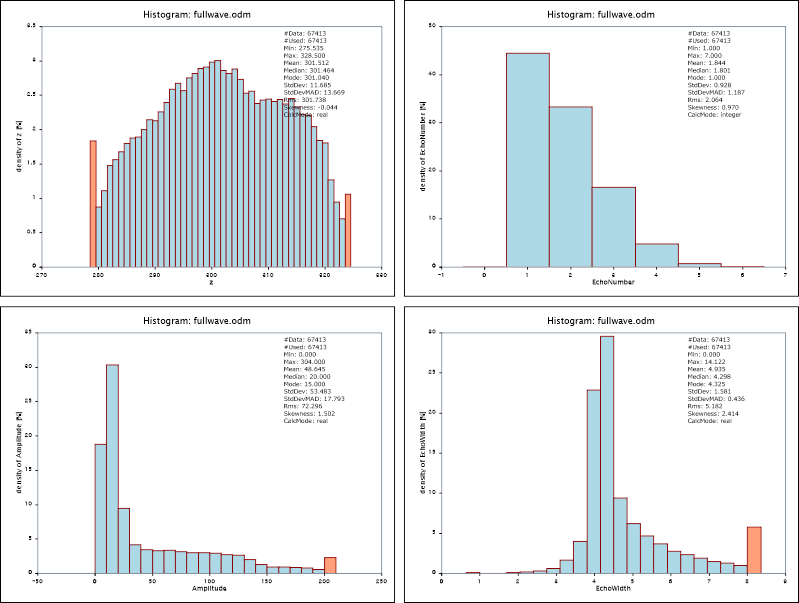Fig. 1: Histograms of ALS point attributes: z (upper left), Echo number (upper right), Amplitude (lower left) and EchoWidth (lower right) A numerical representation of the histogram and statistics can be obtained by specifying an output parameter file as shown in the first example (parameter outParamFile). The resulting XML file histo.xml contains the following output (excerpt): [...] <Module> <Name>opalsHisto</Name> <Version>1.0.0.0</Version> <License>[...] <Parameters> <Parameter Name='inFile'><Val>fullwave.odm</Val></Parameter> <Parameter Name='attribute'><Val>z</Val></Parameter> <Parameter Name='histogram'><Val>Histogram[Label[z] BinWidth[1.000] Bins[(280.989,2984) (281.989,1023) (282.989,1131) (283.989,1157) (284.989,1215) (285.989,1275) (286.989,1324) (287.989,1394) (288.989,1426) (289.989,1502) (290.989,1510) (291.989,1695) (292.989,1819) (293.989,1721) (294.989,1791) (295.989,1933) (296.989,1896) (297.989,1956) (298.989,1958) (299.989,2059) (300.989,1958) (301.989,1901) (302.989,1985) (303.989,1836) (304.989,1799) (305.989,1740) (306.989,1639) (307.989,1652) (308.989,1636) (309.989,1609) (310.989,1648) (311.989,1650) (312.989,1624) (313.989,1604) (314.989,1540) (315.989,1506) (316.989,1421) (317.989,1286) (318.989,1239) (319.989,1156) (320.989,671) (321.989,1544) ] CountData CountUsed Min[275.535] Max[328.500] Mean[301.512] Median[301.464] Mode[300.489] StdDev[11.685] StdDevMAD[13.669] Rms[301.738] Skewness[-0.044] ExcateComputation Quantiles[(0.010,278.430) (0.050,282.238) (0.250,292.189) (0.750,312.619) (0.900,318.009) (0.950,320.754) ]]<Val></Parameter> <Parameter Name='binWidth'><Val>1</Val></Parameter> <Parameter Name='nBins'><Val>50</Val></Parameter> <Parameter Name='plotFile'><Val>fullwave_z_histo.svg</Val></Parameter> </Parameters> </Module> ## Example 2: Raster dataset histogram Example 2 shows how to derive histograms of grid datasets. To generate the underlying grids, please perform the following preprocessing steps: opalsImport -inFile strip11.laz opalsImport -inFile strip21.laz opalsGrid -inFile strip11.odm -outFile strip11.tif -gridSize 1 -interpolation movingPlanes -feature sigmaZ excen opalsGrid -inFile strip21.odm -outFile strip21.tif -gridSize 1 -interpolation movingPlanes -feature sigmaZ excen opalsDiff -inFile strip11.tif strip21.tif -outf diff_11_21.tif opalsAlgebra -inFile strip11_sigmaZ.tif strip11_excen.tif -outf strip11_mask.tif -formula "r && r && r<0.1 && r<0.8" opalsAlgebra -inFile strip21_sigmaZ.tif strip21_excen.tif -outf strip21_mask.tif -formula "r && r && r<0.1 && r<0.8" opalsAlgebra -inFile strip11_mask.tif strip21_mask.tif -outf diff_11_21_mask.tif -formula "r && r ? r*r : invalid" opalsAlgebra -inFile diff_11_21.tif diff_11_21_mask.tif -outf diff_11_21_masked.tif -formula "r && r ? r*r : invalid" This procedure, first, imports the data of strips 19 and 20 (strip11/21.las) into separate ODM files, and generates surface models as well as additional "sigmaZ" (=smoothness) and "excenter" (=extrapolation/occlusion) layers for each strip (strip11.tif, strip11_sigmaZ.tif, strip11_excen.tif). Subsequently, a strip difference model (diff_11_21.tif) and a respective grid mask (diff_11_21_mask.tif) are derived. The examples below (results c.f. Figure 2) show the distribution of the grid heights and excenter layer of the surface model of strip 11. Furthermore, the height differences between strip 11 and 21 are analyzed in two variants, one using all available height differences in the strip overlap area, an the other one considering a grid mask to exclude all rough and occluded pixels. opalsHisto -inFile strip11.tif -nBins 75 opalsHisto -inFile strip11_excen.tif -binwidth 0.1 -sampleRang 0 1 -plotfile strip11_excen_histo.svg opalsHisto -inFile diff_11_21.tif -sampleRange -0.5 0.5 -plotFile diff_11_21_unmasked.svg opalsHisto -inFile diff_11_21_masked.tif -sampleRange -0.5 0.5 -plotFile diff_11_21_masked.svg Please further note, that wild cards are supported in case multiple input datasets.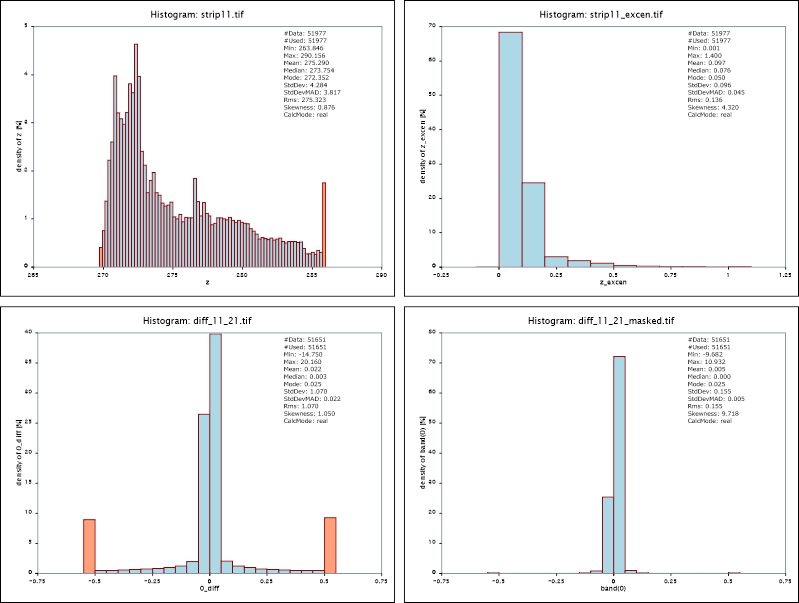Fig. 2: Histograms of grid datasets: DSM heights (upper left), excenter layer (upper right), Unmasked strip differences (lower left), Masked strip differences (lower right) ## Example 3: Standard sample range vs. full sample range By default the module uses the 2% and 98% quantile to limit the histogram. This typically moves outliers to the underflow and overflow bins and presents the relevant data with appropriate resolution. The follow example shows the effect on the z and amplitude attribute for demo dataset strip11. opalsHisto -inf strip11.odm -attr z amplitude -plotfile std_z_histo.svg std_Amplitude_histo.svg opalsHisto -inf strip11.odm -attr z amplitude -samplerange min max -plotfile full_z_histo.svg full_Amplitude_histo.svg Since the dataset contains long ranges and high amplitude values, the full range histograms are not very meaningful (upper images of figure 3) whereas the distribution of the attributes is nicely visable using the standard sample range (lower images of figure 3)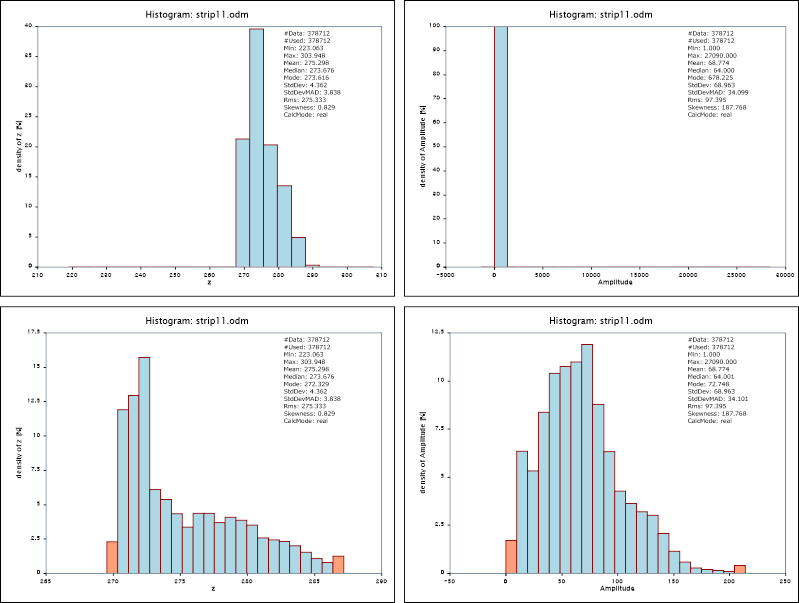Fig. 3: Full range histograms (upper images) and standard sample range using the 2% and 98% quantiles (lower images) ## Example 4: Histogram access via python The main benefit of running Module Histo in Python is that the histogram as well as all standard statistics (min, mean, median, ...) are directly accessible after running the module via the Python API. This is exemplified in the sample script $OPALS_ROOT/demo/histoDemo.py:

1 import opals
2 from opals import Histo, Import
3
4 imp=Import.Import(inFile="fullwave.fwf").run()
5 his=Histo.Histo()
6 his.commons.screenLogLevel = opals.Types.LogLevel.error
7 his.inFile = ["fullwave.odm"]
8 his.probabilities = [0.01,0.05,0.10,0.90,0.95,0.99]
9 his.sampleRange=[280,320]
10 his.binWidth=5
11 his.plotFile="off" # do not create a svg file
12 his.run()
13 stats = his.histogram
14 print("Selected height statistics of dataset: {}".format(his.inFile))
15 print("Min : {:0.2f}".format(stats.getMin()))
16 print("Median: {:0.2f}".format(stats.getMedian()))
17 print("Max : {:0.2f}".format(stats.getMax()))
18 print("q0.95 : {:0.2f}".format(stats.getQuantiles()))
19 print("\nHistogram: lower height bound [m] - abs. #samples : rel. #samples [%]")
20 bAbs, bRel = stats.getAbsBins(),stats.getBins()
21 for i in range(len(bAbs)):
22  print(" {:0.1f} - {:d}: {:0.2f}".format(bAbs[i], bAbs[i], bRel[i]*100.))

To run the script, type:

python histoDemo.py

The script queries the output parameter histogram provided by Module Histo as a complex Python type (class) and uses its access functions to print the min, median, and 95% height quantile of dataset <fullwave.odm>. The following output is printed to the screen:

Selected height statistics of dataset: fullwave.odm
Min : 275.53
Median: 301.46
Max : 328.50
q0.95 : 320.36
Histogram: lower height bound [m] - abs. #samples : rel. #samples [%]
275.0 - 1498 : 2.22
280.0 - 4811 : 7.14
285.0 - 6643 : 9.85
290.0 - 8248 : 12.24
295.0 - 9535 : 14.14
300.0 - 9737 : 14.44
305.0 - 8464 : 12.56
310.0 - 8134 : 12.07
315.0 - 6983 : 10.36
320.0 - 3360 : 4.98

## Example 5: non-continuous histograms

Example 4 demonstrates the skipEmptyBins parameter and its effect on the histogram. For non-continuous (integer) attributes like classification values or the like, a continuous histogram that covers the full data range might be inappropriate since the magnitude of those values is typically irrelevant. Activating the skipEmptyBins parameter as shown below, only plots occupied bins next to each other despite of the bin borders. To make non-continuous histogram easily recognizable, a uniform gab between all bars are inserted.

opalsImport -inFile classify/niederrhein.laz -outFile niederrhein.odm
opalsHisto -inFile niederrhein.odm -attr classification -sampleRange min max -plotFile continuous_histo.svg
opalsHisto -inFile niederrhein.odm -attr classification -sampleRange min max -skipEmptyBins 1 -plotFile non-continuous_histo.svg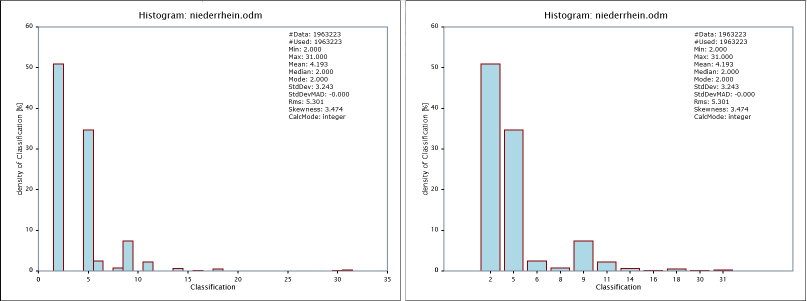Fig. 4: Histogram of classification values in standard, continuous mode (left) and skipEmptyBins, non-continuous mode (right)

The skipEmptyBins parameter not only influences the visual presentation of the histogram but also the actual bin table within the OPALS log as well as the python histogram object.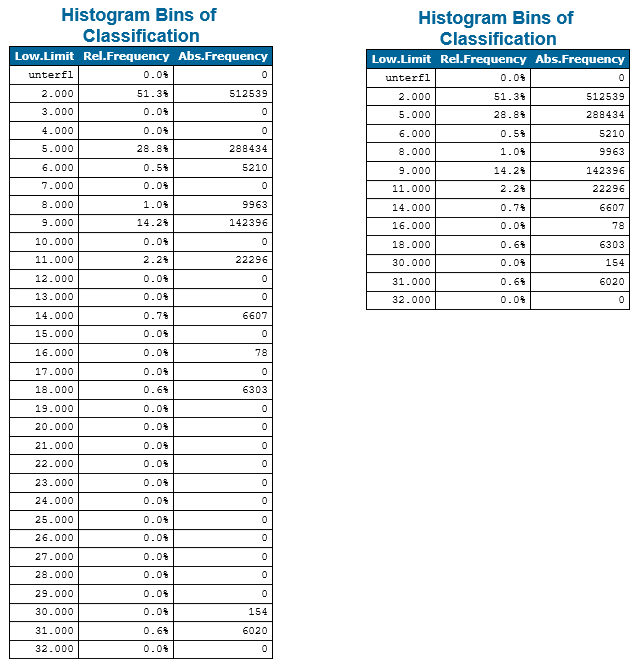Fig. 5: Histogram bins of classification values in standard, continuous mode (left) and skipEmptyBins, non-continuous mode (right)

# References

Ressl, C., Kager, H. and Mandlburger, G., 2008. Quality checking of ALS projects using statistics of strip differences. In: IAPRS, XXXVII, pp. 25399860.

R. Jain and I. Chlamtac, The $$P^2$$ algorithm for dynamic calculation of quantiles and histograms without storing observations, Communications of the ACM, Volume 28 (October), Number 10, 1985, p. 1076-1085.

@ real
allow arbitrary bin width values
@ EchoWidth
Full width at half maximum [ns].
CalcMode
TODO: Enumerator for what?
Definition: calcMode.hpp:8
@ outParamFile
final parameter export
@ nBins
number of different bins (e.g. opalsHisto)
@ binWidth
width of a single bin (e.g. opalsHisto)
@ movingPlanes
moving (tilted) plane interpolation
opalsDiff is the executable file of Module Diff
Definition: ModuleExecutables.hpp:53
opalsAlgebra is the executable file of Module Algebra
Definition: ModuleExecutables.hpp:13
opalsImport is the executable file of Module Import
Definition: ModuleExecutables.hpp:113
@ skipEmptyBins
skips empty bin entries in the historgram (opalsHisto)
@ Amplitude
Linear scale value proportional to the receiving power.
This is the fake namespace of all opals Python scripts.
Definition: doc/temp_doxy/python/__init__.py:1
@ sampleRange
sample (attribute) range (e.g. opalsHisto)
opalsGrid is the executable file of Module Grid
Definition: ModuleExecutables.hpp:93
opalsHisto is the executable file of Module Histo
Definition: ModuleExecutables.hpp:103
@ feature
Use a statistic feature of the boundary gap points for filling.
@ height
surface height
Definition: GridFeature.hpp:25
@ EchoNumber
This is the k-th return/echo for a certain pulse, where for the first return: k==1 (see LAS spec....
@ plotFile
name of plot file (e.g. opalsHisto)
@ formula
formula string for albegraic grid computations (opalsAlgebra)